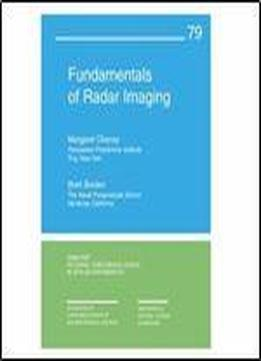# Fundamentals Of Radar Imaging (cbms-nsf Regional Conference Series In Applied Mathematics) by Margaret Cheney / 2009 / English / PDF

Radar imaging is a mathematically rich subject with many ting applications and a large variety of challenging, mathematical open problems. The goal of this book is to provide mathematicians with the background they need to work in the field, building on the foundation of the underlying partial differential equations. The focus is on showing the connection between the physics and the mathematics and on supplying an intuitive mathematical understanding of basic concepts. The book includes a description of how a radar system works, together with the relevant mathematics theory that guides the choice of radar waveforms derivation of the fundamentals of scattering theory derivation and discussion of the image formation process and a long list of current open problems. Readers are assumed to be familiar with the Fourier transform. Other assumed background material includes certain basic facts from vector calculus, complex variables, linear algebra and physics.

views: 571